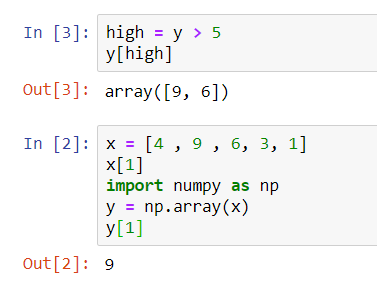# Day 22: Using numpy in Python

Python list has been doing a wonderful job in the as a data collection where it is mutable, able to add, change and delete values from the list. It allows us to hold data with different data types.

numpy package is one of the fundamental package for scientific computing with Python. It is often being used to carry out mathematical operation to entire collections and allows us to perform the computation fast. The installation of the numpy is easily done by going to the command line or the terminal and type the following command:

pip3 install numpy

A simple kick start in the given exercise from the online learning course on using numpy package, it requires us to import the numpy package and use the array. The workaround as below:

```# Create list baseball
baseball = [180, 210, 210, 188, 120, 92, 100, 246]

# Import the numpy package as np
import numpy as np

# Create a numpy array from baseball: np_baseball
np_baseball = np.array(baseball)

# Print out type of np_baseball
print(type(np_baseball))
```

With a random number in a baseball list, then import the numpy and create a new variable, called np_baseball to hold a numpy array and lastly, print out the type of variable, np_baseball. The output of the above code:

```<class 'numpy.ndarray'>
```

### Important notes

Numpy array cannot contain elements with different data types, unlike Python List. If you try to build such list in numpy array, some of the elements’ types are changed to end up with a homogeneous list. It calls coercion.

Secondly, the operator “+”, “-” and etc which we used along with Python List, are now having different meaning for numpy array.

Moving on, there is something quite interesting to be shared here, for numpy package, we can also use boolean numpy arrays. Cool, right? Let’s see the example given by the online learning website, simple one.

```#first set of code, accessing 2nd element using list and numpy array.
#the output is 9.
x = [4 , 9 , 6, 3, 1]
x
import numpy as np
y = np.array(x)
y

#second set of code, check the condition if y's value > 5, and print out the value.
high = y > 5
y[high]
```

I ran the above code in the Jupyter notebook and the result showed as below. I ran the  before .Then in the exercise, I required to create a boolean numpy array which will contain True and False values depend on the condition of player’s BMI < 21 and create a variable to hold the above result. Lastly, print out a numpy array with BMI’s values of all players whose BMI < 21. The keyword here is the usage of square brackets to do a selections on the BMI array.

My source code as below:

```# height and weight are available as a regular lists

# Import numpy
import numpy as np

# Calculate the BMI: bmi
np_height_m = np.array(height_in) * 0.0254
np_weight_kg = np.array(weight_lb) * 0.453592
bmi = np_weight_kg / np_height_m ** 2

# Create the light array
light = np.array(bmi < 21)

# Print out light
print(light)

# Print out BMIs of all baseball players whose BMI is below 21
light = bmi < 21
print(bmi[light])

#output of the 2 prints.
[False False False ..., False False False]
[ 20.54255679  20.54255679  20.69282047  20.69282047  20.34343189
20.34343189  20.69282047  20.15883472  19.4984471   20.69282047
20.9205219 ]
```

Both the y[high] and bmi[light] list the elements that matched the conditions given in the codes.

Summary of the day:

• numpy package
• numpy.array()
• boolean numpy array